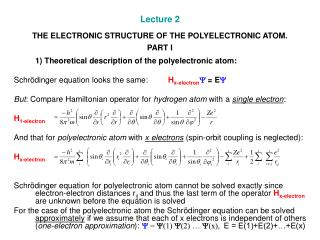Download PresentationLecture 2 THE ELECTRONIC STRUCTURE OF THE POLYELECTRONIC ATOM. PART ILecture 2 THE ELECTRONIC STRUCTURE OF THE POLYELECTRONIC ATOM. PART I - PowerPoint PPT Presentation

Download PresentationLecture 2 THE ELECTRONIC STRUCTURE OF THE POLYELECTRONIC ATOM. PART I
An Image/Link below is provided (as is) to download presentation

Download Policy: Content on the Website is provided to you AS IS for your information and personal use and may not be sold / licensed / shared on other websites without getting consent from its author. While downloading, if for some reason you are not able to download a presentation, the publisher may have deleted the file from their server.

- - - - - - - - - - - - - - - - - - - - - - - - - - - E N D - - - - - - - - - - - - - - - - - - - - - - - - - - -
Presentation Transcript

1. Lecture 2THE ELECTRONIC STRUCTURE OF THE POLYELECTRONIC ATOM. PART I 1) Theoretical description of the polyelectronic atom: Schrödinger equation looks the same: Hx-electronY= EY But: Compare Hamiltonian operator for hydrogen atom with a single electron: H1-electron And that for polyelectronic atom with x electrons (spin-orbit coupling is neglected): Hx-electron Schrödinger equation for polyelectronic atom cannot be solved exactly since electron-electron distances rij and thus the last term of the operator Hx-electron are unknown before the equation is solved For the case of the polyelectronic atom the Schrödinger equation can be solved approximately if we assume that each of x electrons is independent of others (one-electron approximation): Y = Y(1) Y(2) … Y(x), E = E(1)+E(2)+…+E(x)

2. 2) Hydrogen-like orbitals Resulting one-electron wave functions give rise to so-called hydrogen-like orbitals They are of the same shape as corresponding hydrogen atom orbitals, but • Contracted. With increased nuclear charge Z size of atoms decreases along the row of elements with increased Z. So, for 1s orbitals R(r) = • Their energy is a function of both n and l. One-electron energy increases in the order ns < np < nd < nf . The reason for that is electron-electron interactions. (Compare with E = for the hydrogen atom orbitals) • The orbital energy ordering is therefore complex. Energy of the orbitals with quantum number n may be higher than that of the orbitals with quantum number n+1 or even n+2, i.e. 3d > 4s, 4f > 6s etc. There is no uniform ordering of orbital energies for all elements. The following series is very useful though there are exceptions: 1s < 2s < 2p < 3s < 3p < 4s < 3d < 4p < 5s < 4d < 5p < 6s < 5d ≈ 4f < 6p < 7s < 6d < 5f

3. 3) Electron spin and Pauli principle • Each electron has an angular momentum. (An experimental evidence for electron spin: next lecture). Spin quantum number ms is equal to the value of the projection of the electron-spin angular momentumon z-axis and is expressed in units of ħ. ms can have one of two values only, +½ or -½. The fourth, spin quantum number, ms (+½ and -½) is therefore needed to fully characterize the atomic structure. • The electrons with identical set of four quantum numbers, n ,l, ml, ms cannot be found in a given atom (Pauli exclusion principle) • Since there are two allowed values of spin quantum number ms, +½ and -½, each orbital can be occupied by two electrons with opposite spins (one a- and one b-electron).Therefore for a given n the total number of electrons is 2n2. • Each electron has also magnetic moment. The magnitude of the magnetic momentmis given bym = 2.00[s(s+1)]1/2 (here s is spin, s = |ms|) expressed in Bohr magnetons (1 Bohr magneton = 9.27·10‑24 A m2).

4. 4)The Aufbau principle • To find the electron distribution (electron configuration) of a polyelectron atom, the Aufbau principle formalism is used. • According to it, when protons (and neutrons) are added to the nucleus, the electrons are added to orbitals in the sequence in which energies of hydrogen-like orbitals increase: 1s < 2s < 2p < 3s < 3p < 4s < 3d < 4p < 5s < 4d < 5p < 6s < 5d ≈ 4f < 6p < 7s < 6d < 5f Electronic configuration of the elements: http://chemistry.about.com/library/weekly/aa013103a.htm

5. 5)Filled shells and relativistic effects • There are no exceptions from the rule for the elements H-V. • Some exceptions which occur for Cr and some other heavier elements (Cu, Nb, Mo, Ru-Ag and also 4f and 5f-block elements) are due to: a) the increased stability of half- or completely filledd-shells (Cr, Cu, Mo, Pd, Ag); b) the relativisticradial expansion and energetic destabilization of the outer 5d-, 6d and 5f-shells (i.e. compare Ta – Pt with Nb – Pd).

6. Summary • The Schrödinger equation for multyelectron atoms cannot be solved directly. One-electron approximation and SCF procedure are used usually to solve it • Resulting hydrogen-like orbitals are contracted and follow a different energy order as compared with hydrogen orbitals • Fourth quantum number ms is required to completely describe multielectron systems. Pauli exclusion principle is applied • The Aufbau principle allows to determine (approximate) electronic configuration of polyelectronic atoms; exceptions are due to increased stability of half- or completely filledshells and the relativisticradial expansion and energetic destabilization of the outer 5d- and 5f-shells